## Module contents¶

Core of the line-by-line calculations

class LevelsList(parfunc, bands, levelsfmt, sortby='Ei', copy_lines=False, verbose=True)[source]

Bases: object

A class to generate a Spectrum from a list of precalculated bands at a given reference temperature.

Warning

only valid under optically thin conditions!!

E_bands[source]
Qref[source]
Qvib_ref[source]
Trot_ref[source]
Tvib_ref[source]
bands[source]
bands_ref[source]
copy_lines[source]
cum_weight[source]
eq_spectrum(Tgas, overpopulation=None, mole_fraction=None, path_length=None, save_rescaled_bands=False)[source]

Warning

only valid under optically thin conditions!!

Parameters
• … same as usually. If None, then the reference value (used to

• calculate bands) is used

Other Parameters

save_rescaled_bands (boolean) – save updated bands. Take some time as it requires rescaling all bands individually (which is only done on the MergedSlabs usually) Default False

levelsfmt[source]
lvl_index[source]
mole_fraction_ref[source]
non_eq_spectrum(Tvib=None, Trot=None, Ttrans=None, vib_distribution='boltzmann', overpopulation=None, mole_fraction=None, path_length=None, save_rescaled_bands=False)[source]

Warning

only valid under optically thin conditions!!

Parameters
• … same as usually. If None, then the reference value (used to

• calculate bands) is used

Other Parameters

save_rescaled_bands (boolean) – save updated bands. Take some time as it requires rescaling all bands individually (which is only done on the MergedSlabs usually) Default False

Notes

Implementation:

Generation of a new spectrum is done by recombination of the precalculated bands with

parfunc[source]
path_length_ref[source]
plot_vib_populations(nfig=None, **kwargs)[source]

Plot current distribution of vibrational levels.

By constructions populations are shown as divided by the state degeneracy, i.e, g = gv * (2J+1) * gi * gs

Parameters
• nfig (str, int) – name of Figure to plot on

• kwargs (**dict) – arguments are forwarded to plot()

sorted_bands[source]
verbose[source]
vib_distribution_ref[source]
vib_levels[source]
class SpectrumFactory(wmin=None, wmax=None, wunit=cm - 1, wavenum_min=None, wavenum_max=None, wavelength_min=None, wavelength_max=None, Tref=296, pressure=1.01325, mole_fraction=1, path_length=1, wstep=0.01, molecule=None, isotope='all', medium='air', truncation=50, neighbour_lines=0, pseudo_continuum_threshold=0, self_absorption=True, chunksize=None, optimization='simple', folding_thresh=1e-06, zero_padding=-1, broadening_method='voigt', cutoff=0, parsum_mode='full summation', verbose=True, warnings=True, save_memory=False, export_populations=None, export_lines=False, emulate_gpu=False, **kwargs)[source]

Bases: BandFactory

A class to put together all functions related to loading CDSD / HITRAN databases, calculating the broadenings, and summing over all the lines.

Parameters
• wmin, wmax (float or Quantity) – a hybrid parameter which can stand for minimum (maximum) wavenumber or minimum (maximum) wavelength depending upon the unit accompanying it. If dimensionless, wunit is considered as the accompanying unit.

• wunit ('nm', 'cm-1') – the unit accompanying wmin and wmax. Can only be passed with wmin and wmax. Default is "cm-1".

• wavenum_min, wavenum_max (float(cm^-1) or Quantity) – minimum (maximum) wavenumber to be processed in $$cm^{-1}$$. use astropy.units to specify arbitrary inverse-length units.

• wavelength_min, wavelength_max (float(nm) or Quantity) – minimum (maximum) wavelength to be processed in $$nm$$. This wavelength can be in 'air' or 'vacuum' depending on the value of the parameter medium=. use astropy.units to specify arbitrary length units.

• pressure (float(bar) or Quantity) – partial pressure of gas in bar. Default 1.01325 (1 atm). use astropy.units to specify arbitrary pressure units. For example, 1013.25 * u.mbar.

• mole_fraction (float [ 0 - 1]) – species mole fraction. Default 1. Note that the rest of the gas is considered to be air for collisional broadening.

• path_length (float(cm) or Quantity) – path length in cm. Default 1. use astropy.units to specify arbitrary length units.

• molecule (int, str, or None) – molecule id (HITRAN format) or name. If None, the molecule can be infered from the database files being loaded. See the list of supported molecules in MOLECULES_LIST_EQUILIBRIUM and MOLECULES_LIST_NONEQUILIBRIUM. Default None.

• isotope (int, list, str of the form '1,2', or 'all') – isotope id (sorted by relative density: (eg: 1: CO2-626, 2: CO2-636 for CO2). See HITRAN documentation for isotope list for all species. If ‘all’, all isotopes in database are used (this may result in larger computation times!). Default 'all'

• medium ('air', 'vacuum') – propagating medium when giving inputs with 'wavenum_min', 'wavenum_max'. Does not change anything when giving inputs in wavenumber. Default 'air'

Other Parameters
• Tref (K) – Reference temperature for calculations (linestrength temperature correction). HITRAN database uses 296 Kelvin. Default 296 K

• self_absorption (boolean) – Compute self absorption. If False, spectra are optically thin. Default True.

• truncation (float ($$cm^{-1}$$)) – Half-width over which to compute the lineshape, i.e. lines are truncated on each side after truncation ($$cm^{-1}$$) from the line center. If None, use no truncation (lineshapes spread on the full spectral range). Default is 300 $$cm^{-1}$$

Note

Large values (> 50) can induce a performance drop (computation of lineshape typically scale as $$~truncation ^2$$ ). The default 300 was chosen to maintain a good accuracy, and still exhibit the sub-Lorentzian behavior of most lines far (few hundreds $$cm^{-1}$$) from the line center.

• neighbour_lines (float ($$cm^{-1}$$)) – The calculated spectral range is increased (by neighbour_lines cm-1 on each side) to take into account overlaps from out-of-range lines. Default is 0 $$cm^{-1}$$.​

• wstep (float (cm-1) or 'auto') – Resolution of wavenumber grid. Default 0.01 cm-1. If 'auto', it is ensured that there are slightly more points for each linewidth than the value of "GRIDPOINTS_PER_LINEWIDTH_WARN_THRESHOLD" in radis.config (~/radis.json)

Note

wstep = ‘auto’ is optimized for performances while ensuring accuracy, but is still experimental in 0.9.30. Feedback welcome!

• cutoff (float (~ unit of Linestrength: cm-1/(#.cm-2))) – discard linestrengths that are lower that this, to reduce calculation times. 1e-27 is what is generally used to generate databases such as CDSD. If 0, no cutoff. Default 1e-27.

• parsum_mode (‘full summation’, ‘tabulation’) – how to compute partition functions, at nonequilibrium or when partition function are not already tabulated. 'full summation' : sums over all (potentially millions) of rovibrational levels. 'tabulation' : builds an on-the-fly tabulation of rovibrational levels (500 - 4000x faster and usually accurate within 0.1%). Default full summation'

Note

parsum_mode= ‘tabulation’ is new in 0.9.30, and makes nonequilibrium calculations of small spectra extremelly fast. Will become the default after 0.9.31.

• pseudo_continuum_threshold (float) – if not 0, first calculate a rough approximation of the spectrum, then moves all lines whose linestrength intensity is less than this threshold of the maximum in a semi-continuum. Values above 0.01 can yield significant errors, mostly in highly populated areas. 80% of the lines can typically be moved in a continuum, resulting in 5 times faster spectra. If 0, no semi-continuum is used. Default 0.

• save_memory (boolean) – if True, removes databases calculated by intermediate functions (for instance, delete the full database once the linestrength cutoff criteria was applied). This saves some memory but requires to reload the database & recalculate the linestrength for each new parameter. Default False.

• export_populations ('vib', 'rovib', None) – if not None, store populations in Spectrum. Either store vibrational populations (‘vib’) or rovibrational populations (‘rovib’). Default None

• export_lines (boolean) – if True, saves details of all calculated lines in Spectrum. This is necessary to later use line_survey(), but can take some space. Default False.

• chunksize (int, or None) – Splits the lines database in several chuncks during calculation, else the multiplication of lines over all spectral range takes too much memory and slows the system down. Chunksize let you change the default chunck size. If None, all lines are processed directly. Usually faster but can create memory problems. Default None

• optimization ("simple", "min-RMS", None) – If either "simple" or "min-RMS" LDM optimization for lineshape calculation is used: - "min-RMS" : weights optimized by analytical minimization of the RMS-error (See: [Spectral-Synthesis-Algorithm]) - "simple" : weights equal to their relative position in the grid

If using the LDM optimization, broadening method is automatically set to 'fft'. If None, no lineshape interpolation is performed and the lineshape of all lines is calculated.

Refer to [Spectral-Synthesis-Algorithm] for more explanation on the LDM method for lineshape interpolation.

Default "min-RMS"

• folding_thresh (float) – Folding is a correction procedure thet is applied when the lineshape is calculated with the fft broadening method and the linewidth is comparable to wstep, that prevents sinc(v) modulation of the lineshape. Folding continues until the lineshape intensity is below folding_threshold. Setting to 1 or higher effectively disables folding correction.

Range: 0.0 < folding_thresh <= 1.0 Default: 1e-6

• zero_padding (int) – Zero padding is used in conjunction with the fft broadening method to prevent circular convolution at the cost of performance. When set to -1, padding is set equal to the spectrum length, which guarantees a linear convolution.

Range: 0 <= zero_padding <= len(w), or zero_padding = -1 Default: -1

• broadening_method ("voigt", "convolve", "fft") – Calculates broadening with a direct voigt approximation (‘voigt’) or by convoluting independantly calculated Doppler and collisional broadening (‘convolve’). First is much faster, 2nd can be used to compare results. This SpectrumFactory parameter can be manually adjusted a posteriori with:

sf = SpectrumFactory(...)


Fast fourier transform 'fft' is only available if using the LDM lineshape calculation optimization. Because the LDM convolves all lines at the same time, and thus operates on large arrays, 'fft' becomes more appropriate than convolutions in real space ('voit', 'convolve' )

By default, use "fft" for any optimization, and "voigt" if optimization is None .

• warnings (bool, or one of ['warn', 'error', 'ignore'], dict) – If one of ['warn', 'error', 'ignore'], set the default behaviour for all warnings. Can also be a dictionary to set specific warnings only. Example:

warnings = {'MissingSelfBroadeningWarning':'ignore',
'NegativeEnergiesWarning':'ignore',
'HighTemperatureWarning':'ignore'}


See default_warning_status for more information.

• verbose (boolean, or int) – If False, stays quiet. If True, tells what is going on. If >=2, gives more detailed messages (for instance, details of calculation times). Default True.

Examples

An example using SpectrumFactory, load_databank(), the Spectrum methods, and units

from radis import SpectrumFactory
from astropy import units as u
sf = SpectrumFactory(wavelength_min=4165 * u.nm,
wavelength_max=4200 * u.nm,
isotope='1,2',
truncation=10,  # cm-1
optimization=None,
medium='vacuum',
verbose=1,    # more for more details
)
s = sf.eq_spectrum(Tgas=300 * u.K, path_length=1 * u.cm)
s.rescale_path_length(0.01)    # cm


Refer to the online Examples for more cases.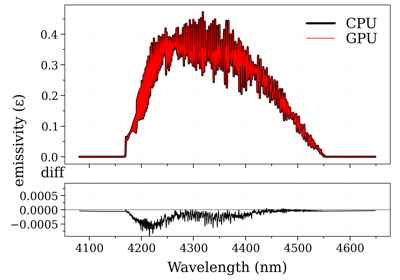GPU Accelerated Spectra

GPU Accelerated Spectra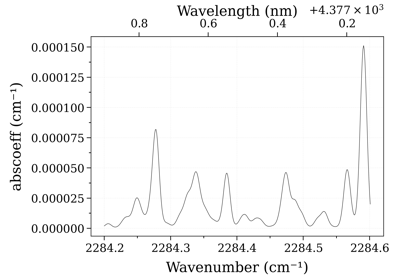Use Custom Abundances

Use Custom Abundances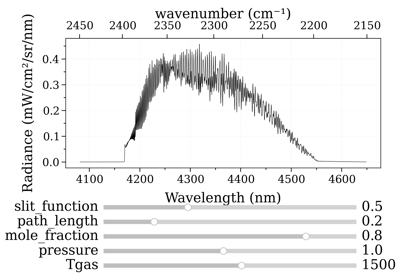Real-time GPU Accelerated Spectra (Interactive)

Real-time GPU Accelerated Spectra (Interactive)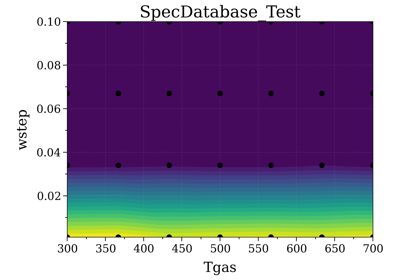Spectrum Database

Spectrum DatabaseMulti-temperature Fit

Multi-temperature Fit1 temperature fit

1 temperature fit

NotesHigh-level wrapper to SpectrumFactory:

Main Methods:

Inputs and parameters can be accessed a posteriori with :

eq_spectrum(Tgas, mole_fraction=None, path_length=None, pressure=None, name=None) [source]

Generate a spectrum at equilibrium.

Parameters
• Tgas (float or Quantity) – Gas temperature (K)

• mole_fraction (float) – database species mole fraction. If None, Factory mole fraction is used.

• path_length (float or Quantity) – slab size (cm). If None, the default Factory path_length is used.

• pressure (float or Quantity) – pressure (bar). If None, the default Factory pressure is used.

• name (str) – output Spectrum name (useful in batch)

Returns

s

Returns a Spectrum object

Use the get() method to get something among ['radiance', 'radiance_noslit', 'absorbance', etc...]

Or directly the plot() method to plot it. See _ to get an overview of all Spectrum methods

Return type

Spectrum

Examples

::

from radis import SpectrumFactory sf = SpectrumFactory( wavenum_min=2900, wavenum_max=3200, molecule=”OH”, wstep=0.1, ) sf.fetch_databank(“hitemp”)

s1 = sf.eq_spectrum(Tgas=300, path_length=1, pressure=0.1) s2 = sf.eq_spectrum(Tgas=500, path_length=1, pressure=0.1)

References

1

RADIS doc: Spectrum how to?

eq_spectrum_gpu(Tgas, mole_fraction=None, path_length=None, pressure=None, name=None, emulate=False) [source]

Generate a spectrum at equilibrium with calculation of lineshapes and broadening done on the GPU.

Note

This method requires CUDA compatible hardware to execute. For more information on how to setup your system to run GPU-accelerated methods using CUDA and Cython, check GPU Spectrum Calculation on RADIS

Parameters
• Tgas (float or Quantity) – Gas temperature (K)

• mole_fraction (float) – database species mole fraction. If None, Factory mole fraction is used.

• path_length (float or Quantity) – slab size (cm). If None, the default Factory path_length is used.

• pressure (float or Quantity) – pressure (bar). If None, the default Factory pressure is used.

• name (str) – output Spectrum name (useful in batch)

Other Parameters

emulate (bool) – if True, execute the GPU code on the CPU (useful for development)

Returns

s

Returns a Spectrum object

Use the get() method to get something among ['radiance', 'radiance_noslit', 'absorbance', etc...]

Or directly the plot() method to plot it. See _ to get an overview of all Spectrum methods

Return type

Spectrum

ExamplesGPU Accelerated Spectra

GPU Accelerated SpectraReal-time GPU Accelerated Spectra (Interactive)

Real-time GPU Accelerated Spectra (Interactive)
eq_spectrum_gpu_interactive(var='transmittance', slit_FWHM=0.0, plotkwargs={}, *vargs, **kwargs) [source]
Parameters
Returns

s – DESCRIPTION.

Return type

TYPE

Examples

from radis import SpectrumFactory
from radis.tools.plot_tools import ParamRange

sf = SpectrumFactory(2200, 2400, # cm-1
molecule='CO2',
isotope='1,2,3',
wstep=0.002,
)

sf.fetch_databank('hitemp')

s = sf.eq_spectrum_gpu_interactive(Tgas=ParamRange(300.0,2000.0,1200.0), #K
pressure=0.2, #bar
mole_fraction=0.1,
path_length=ParamRange(0,10,2.0), #cm
slit_FWHM=ParamRange(0,1,0), #cm
emulate=False,  # if True, execute the GPU code on the CPU
)

fit_spectrum(s_exp, model, fit_parameters, bounds={}, plot=False, solver_options={'maxiter': 300}, **kwargs) Union[Spectrum, OptimizeResult][source]

Fit an experimental spectrum with an arbitrary model and an arbitrary number of fit parameters.

Parameters
• s_exp (Spectrum) – experimental spectrum. Should have only spectral array only. Use take(), e.g:

sf.fit_spectrum(s_exp.take('transmittance'))

• model (func -> Spectrum) – a line-of-sight model returning a Spectrum. Example : Tvib12Tvib3Trot_NonLTEModel()

• fit_parameters (dict) –

example:

{fit_parameter:initial_value}

• bounds (dict, optional) –

example:

{fit_parameter:[min, max]}

• fixed_parameters (dict) –

fixed parameters given to the model. Example:

fit_spectrum(fixed_parameters={"vib_distribution":"treanor"})

Other Parameters
• plot (bool) – if True, plot spectra as they are computed; and plot the convergence of the residual. Default False

• solver_options (dict) – parameters forwarded to the solver. More info in minimize Example:

{"maxiter": (int)  max number of iteration default 300,
}

• kwargs (dict) – forwarded to fit_spectrum()

Returns

ExamplesMulti-temperature Fit

Multi-temperature Fit1 temperature fit

1 temperature fit

More advanced tools for interactive fitting of multi-dimensional, multi-slabs spectra can be found in fitroom.

generate_perf_profile()[source]

Generate a visual/interactive performance profile diagram for the last calculated spectrum, with tuna.

Requires a profiler key with in Spectrum.conditions, and tuna installed.

Note

print_perf_profile() is an ascii-version which does not require tuna.

Examples

sf = SpectrumFactory(...)
sf.eq_spectrum(...)
sf.generate_perf_profile()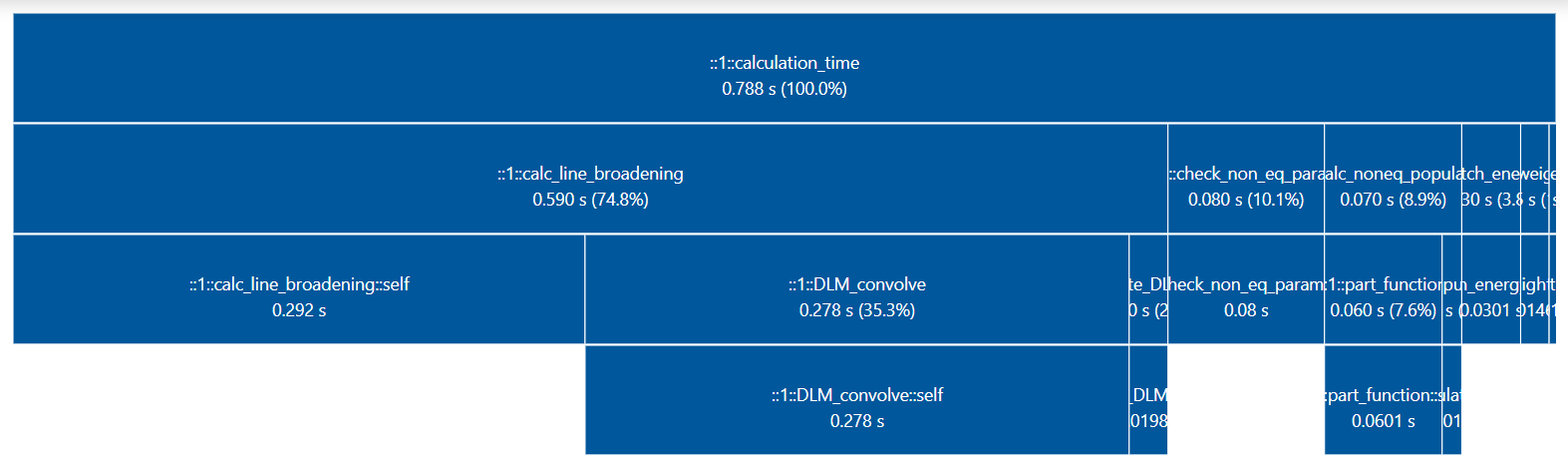Note

You can also profile with tuna directly:

python -m cProfile -o program.prof your_radis_script.py

non_eq_spectrum(Tvib, Trot, Ttrans=None, mole_fraction=None, path_length=None, pressure=None, vib_distribution='boltzmann', rot_distribution='boltzmann', overpopulation=None, name=None) [source]

Calculate emission spectrum in non-equilibrium case. Calculates absorption with broadened linestrength and emission with broadened Einstein coefficient.

Parameters
• Tvib (float) – vibrational temperature [K] can be a tuple of float for the special case of more-than-diatomic molecules (e.g: CO2)

• Trot (float) – rotational temperature [K]

• Ttrans (float) – translational temperature [K]. If None, translational temperature is taken as rotational temperature (valid at 1 atm for times above ~ 2ns which is the RT characteristic time)

• mole_fraction (float) – database species mole fraction. If None, Factory mole fraction is used.

• path_length (float or Quantity) – slab size (cm). If None, the default Factory path_length is used.

• pressure (float or Quantity) – pressure (bar). If None, the default Factory pressure is used.

Other Parameters
• vib_distribution ('boltzmann', 'treanor') – vibrational distribution

• rot_distribution ('boltzmann') – rotational distribution

• overpopulation (dict, or None) –

add overpopulation factors for given levels:

{level:overpopulation_factor}

• name (str) – output Spectrum name (useful in batch)

Returns

s

Returns a Spectrum object

Use the get() method to get something among ['radiance', 'radiance_noslit', 'absorbance', etc...]

Or directly the plot() method to plot it. See _ to get an overview of all Spectrum methods

Return type

Spectrum

Examples

from radis import SpectrumFactory
sf = SpectrumFactory(
wavenum_min=2000,
wavenum_max=3000,
molecule="CO",
isotope="1,2,3",
wstep="auto"
)

s1 = sf.non_eq_spectrum(Tvib=2000, Trot=600, path_length=1, pressure=0.1)
s2 = sf.non_eq_spectrum(Tvib=2000, Trot=600, path_length=1, pressure=0.1)


Multi-vibrational temperature. Below we compare non-LTE spectra of CO2 where all vibrational temperatures are equal, or where the bending & symmetric modes are in equilibrium with rotation

from radis import SpectrumFactory
sf = SpectrumFactory(
wavenum_min=2000,
wavenum_max=3000,
molecule="CO2",
isotope="1,2,3",
)
# nonequilibrium between bending+symmetric and asymmetric modes :
s1 = sf.non_eq_spectrum(Tvib=(600, 600, 2000), Trot=600, path_length=1, pressure=1)
# all vibrational temperatures are equal :
s2 = sf.non_eq_spectrum(Tvib=(2000, 2000, 2000), Trot=600, path_length=1, pressure=1)


References

1

RADIS doc: Spectrum how to?

optically_thin_power(Tgas=None, Tvib=None, Trot=None, Ttrans=None, vib_distribution='boltzmann', rot_distribution='boltzmann', mole_fraction=None, path_length=None, unit='mW/cm2/sr')[source]

Calculate total power emitted in equilibrium or non-equilibrium case in the optically thin approximation: it sums all emission integral over the total spectral range.

Warning

this is a fast implementation that doesnt take into account the contribution of lines outside the given spectral range. It is valid for spectral ranges surrounded by no lines, and spectral ranges much broaded than the typical line broadening (~ 1-10 cm-1 in the infrared)

If what you’re looking for is an accurate simulation on a narrow spectral range you better calculate the spectrum (that does take all of that into account) and integrate it with get_power()

Parameters
• Tgas (float) – equilibrium temperature [K] If doing a non equilibrium case it should be None. Use Ttrans for translational temperature

• Tvib (float) – vibrational temperature [K]

• Trot (float) – rotational temperature [K]

• Ttrans (float) – translational temperature [K]. If None, translational temperature is taken as rotational temperature (valid at 1 atm for times above ~ 2ns which is the RT characteristic time)

• mole_fraction (float) – database species mole fraction. If None, Factory mole fraction is used.

• path_length (float) – slab size (cm). If None, Factory mole fraction is used.

• unit (str) – output unit. Default 'mW/cm2/sr'

Returns

float – see unit=.

Return type

Returns total power density in mW/cm2/sr (unless different unit is chosen),

predict_time()[source]

predict_time(self) uses the input parameters like Spectral Range, Number of lines, wstep, truncation to predict the estimated calculation time for the Spectrum broadening step(bottleneck step) for the current optimization and broadening_method. The formula for predicting time is based on benchmarks performed on various parameters for different optimization, broadening_method and deriving its time complexity.

Complexity vs Calculation Time Visualizations LBL>Voigt: LINK, DIT>Voigt: LINK, DIT>FFT: LINK

Returns

float

Return type

Predicted time in seconds.

print_perf_profile(number_format='{:.3f}', precision=16)[source]

Prints Profiler output dictionary in a structured manner for the last calculated spectrum

Examples

sf.print_perf_profile()

# output >>
spectrum_calculation      0.189s ████████████████
check_line_databank              0.000s
check_non_eq_param               0.042s ███
fetch_energy_5                   0.015s █
calc_weight_trans                0.008s
reinitialize                     0.002s
copy_database                    0.000s
memory_usage_warning             0.002s
reset_population                 0.000s
calc_noneq_population            0.041s ███
part_function                    0.035s ██
population                       0.006s
scaled_non_eq_linestrength       0.005s
map_part_func                    0.001s
corrected_population_se          0.003s
calc_emission_integral           0.006s
applied_linestrength_cutoff      0.002s
calc_lineshift                   0.001s
calc_hwhm                        0.007s
generate_wavenumber_arrays       0.001s
precompute_LDM_lineshapes        0.012s
LDM_Initialized_vectors          0.000s
LDM_closest_matching_line        0.001s
LDM_Distribute_lines             0.001s
LDM_convolve                     0.060s █████
others                           0.001s
calc_other_spectral_quan         0.003s
generate_spectrum_obj            0.000s
others                           -0.016s

Other Parameters

precision (int, optional) – total number of blocks. Default 16.

calc_spectrum(wmin=None, wmax=None, wunit=cm - 1, Tgas=None, Tvib=None, Trot=None, pressure=1.01325, molecule=None, isotope='all', mole_fraction=1, path_length=1, databank='hitran', medium='air', wstep=0.01, truncation=50, neighbour_lines=0, cutoff=1e-27, parsum_mode='full summation', optimization='simple', chunksize=None, broadening_method='voigt', overpopulation=None, name=None, save_to='', use_cached=True, mode='cpu', export_lines=False, verbose=True, return_factory=False, **kwargs) [source]

Calculate a Spectrum.

Can automatically download databases (HITRAN/HITEMP) or use manually downloaded local databases, under equilibrium or non-equilibrium, with or without overpopulation, using either CPU or GPU.

It is a wrapper to SpectrumFactory class. For advanced used, please refer to the aforementionned class.

Parameters
• wmin, wmax (float [$$cm^{-1}$$] or Quantity) –

wavelength/wavenumber range. If no units are given, use $$cm^{-1}$$

calc_spectrum(2000, 2300, ... )   # cm-1
calc_spectrum(4000, 4200, wunit='nm', ...)


You can use arbitrary units:

import astropy.units as u
calc_spectrum(2.5*u.um, 3.0*u.um, ...)

• wunit ('nm', 'cm-1') – unit for wmin and wmax. Default is "cm-1".

• Tgas (float [$$K$$]) – Gas temperature. If non equilibrium, is used for $$T_{translational}$$. Default 300 K​

• Tvib, Trot (float [$$K$$]) – Vibrational and rotational temperatures (for non-LTE calculations). If None, they are at equilibrium with Tgas ​.

• pressure (float [$$bar$$] or Quantity) –

partial pressure of gas in bar. Default 1.01325 (1 atm)​. Use arbitrary units:

import astropy.units as u
calc_spectrum(..., pressure=20*u.mbar)

• molecule (int, str, list or None) – molecule id (HITRAN format) or name. For multiple molecules, use a list. The 'isotope', 'mole_fraction', 'databank' and 'overpopulation' parameters must then be dictionaries. If None, the molecule can be infered from the database files being loaded. See the list of supported molecules in MOLECULES_LIST_EQUILIBRIUM and MOLECULES_LIST_NONEQUILIBRIUM. Default None.​

• isotope (int, list, str of the form '1,2', or 'all', or dict) – isotope id (sorted by relative density: (eg: 1: CO2-626, 2: CO2-636 for CO2). See [HITRAN-2020] documentation for isotope list for all species. If 'all', all isotopes in database are used (this may result in larger computation times!). Default 'all'.

For multiple molecules, use a dictionary with molecule names as keys

isotope={'CO2':'1,2' ,  'CO':'1,2,3' }​

• mole_fraction (float or dict) – database species mole fraction. Default 1.

For multiple molecules, use a dictionary with molecule names as keys

mole_fraction={'CO2': 0.8, 'CO':0.2}​

• path_length (float [$$cm$$] or Quantity) –

slab size. Default 1 cm​. Use arbitrary units:

import astropy.units as u
calc_spectrum(..., path_length=1000*u.km)

• databank (str or dict) – can be either: - 'hitran', to fetch the latest HITRAN version

through fetch_hitran() (download full database with [HAPI]) or fetch_astroquery() (download only the required range). To use one mode or the other, use

databank=('hitran', 'full')     # download and cache full database, all isotopes
databank=('hitran', 'range')    # download and cache required range, required isoope

• 'hitemp', to fetch the latest HITEMP version through fetch_hitemp(). Downloads all lines and all isotopes.

• 'exomol', to fetch the latest ExoMol database through fetch_exomol(). To download a specific database use (more info in fetch_exomol)

databank=('exomol', 'EBJT')   # 'EBJT' is a specific ExoMol database name

• 'geisa', to fetch the GEISA 2020 database through fetch_geisa(). Downloads all lines and all isotopes.

• the name of a a valid database file, in which case the format is inferred. For instance, '.par' is recognized as hitran/hitemp format. Accepts wildcards '*' to select multiple files

databank='PATH/TO/co_*.par'

• the name of a spectral database registered in your ~/radis.json configuration file

databank='MY_SPECTRAL_DATABASE'


Default 'hitran'. See DatabankLoader for more information on line databases, and DBFORMAT for your ~/radis.json file format.

For multiple molecules, use a dictionary with molecule names as keys:

databank='hitran'     # automatic download (or 'hitemp')
databank='PATH/TO/05_HITEMP2019.par'    # path to a file
databank='*CO2*.par' #to get all the files that have CO2 in their names (case insensitive)
databank='HITEMP-2019-CO'   # user-defined database in Configuration file
databank = {'CO2' : 'PATH/TO/05_HITEMP2019.par', 'CO' : 'hitran'}  # for multiple molecules

Other Parameters
• medium ('air', 'vacuum') – propagating medium when giving inputs with 'wavenum_min', 'wavenum_max'. Does not change anything when giving inputs in wavenumber. Default ’air’

• wstep (float ($$cm^{-1}$$) or 'auto') – Resolution of wavenumber grid. Default 0.01 cm-1. If 'auto', it is ensured that there are slightly more or less than radis.config ['GRIDPOINTS_PER_LINEWIDTH_WARN_THRESHOLD'] points for each linewidth.

Note

wstep = ‘auto’ is optimized for performances while ensuring accuracy, but is still experimental in 0.9.30. Feedback welcome!

• truncation (float ($$cm^{-1}$$)) – Half-width over which to compute the lineshape, i.e. lines are truncated on each side after truncation ($$cm^{-1}$$) from the line center. If None, use no truncation (lineshapes spread on the full spectral range). Default is 300 $$cm^{-1}$$

Note

Large values (> 50) can induce a performance drop (computation of lineshape typically scale as $$~truncation ^2$$ ). The default 300 was chosen to maintain a good accuracy, and still exhibit the sub-Lorentzian behavior of most lines far (few hundreds $$cm^{-1}$$) from the line center.

• neighbour_lines (float ($$cm^{-1}$$)) – The calculated spectral range is increased (by neighbour_lines cm-1 on each side) to take into account overlaps from out-of-range lines. Default is 0 $$cm^{-1}$$.​

• cutoff (float (~ unit of Linestrength: $$cm^{-1}/(molec.cm^{-2})$$)) – discard linestrengths that are lower that this, to reduce calculation times. 1e-27 is what is generally used to generate line databases such as CDSD. If 0, no cutoff. Default 1e-27 .

• parsum_mode (‘full summation’, ‘tabulation’) – how to compute partition functions, at nonequilibrium or when partition function are not already tabulated. 'full summation' : sums over all (potentially millions) of rovibrational levels. 'tabulation' : builds an on-the-fly tabulation of rovibrational levels (500 - 4000x faster and usually accurate within 0.1%). Default 'full summation'

Note

parsum_mode= ‘tabulation’ is new in 0.9.30, and makes nonequilibrium calculations of small spectra extremelly fast. Will become the default after 0.9.31.

• optimization ("simple", "min-RMS", None) – If either "simple" or "min-RMS" LDM optimization for lineshape calculation is used:

• "min-RMS" : weights optimized by analytical minimization of the RMS-error (See: [Spectral-Synthesis-Algorithm])

• "simple" : weights equal to their relative position in the grid

If using the LDM optimization, broadening method is automatically set to 'fft'. If None, no lineshape interpolation is performed and the lineshape of all lines is calculated. Refer to [Spectral-Synthesis-Algorithm] for more explanation on the LDM method for lineshape interpolation. Default "simple".

• overpopulation (dict) – dictionary of overpopulation compared to the given vibrational temperature. Default None. Example:

overpopulation = {'CO2' : {'(0000)->(0001)': 2.5,
'(0001)->(0002)': 1,
'(0110)->(0111)': 1,
'(0111)->(0112)': 1 }
}​

• export_lines (boolean) – if True, saves details of all calculated lines in Spectrum. This is necessary to later use line_survey(), but can take some space. Default False.

• name (str) – name of the output Spectrum. If None, a unique ID is generated.

• save_to (str) – save to a spec file which contains absorption & emission features, all calculation parameters, and can be opened with load_spec(). File can be reloaded and exported to text formats afterwards, see savetxt(). If file already exists, replace.

• use_cached (boolean) – use cached files for line database and energy database. Default True.​

• verbose (boolean, or int) – If False, stays quiet. If True, tells what is going on. If >=2, gives more detailed messages (for instance, details of calculation times). Default True.​

• mode ('cpu', 'gpu', 'emulated_gpu') – if set to 'cpu', computes the spectra purely on the CPU. if set to 'gpu', offloads the calculations of lineshape and broadening steps to the GPU making use of parallel computations to speed up the process. Default 'cpu'. Note that mode='gpu' requires CUDA compatible hardware to execute. For more information on how to setup your system to run GPU-accelerated methods using CUDA and Cython, check GPU Spectrum Calculation on RADIS To try the GPU code without an actual GPU, you can use mode='emulated_gpu'. This will run the GPU equivalent code on the CPU.

• return_factory (bool) – if True, return the SpectrumFactory that computes the spectrum. Useful to access computational parameters, the line database, or to start batch-computations from a first spectrum calculation. Ex:

s, sf = calc_spectrum(..., return_factory=True)
sf.df1  # see the lines calculated
sf.eq_spectrum(...)  #  new calculation without reloading the database

• **kwargs (other inputs forwarded to SpectrumFactory) – For instance: warnings. See SpectrumFactory documentation for more details on input.

Returns

• Spectrum – Output spectrum:

• Use the get() method to retrieve a spectral quantity ('radiance', 'radiance_noslit', 'absorbance', etc…)

• Or the plot() method to plot it directly.

• See _ to get an overview of all Spectrum methods

• :py:class:~radis.lbl.factory.SpectrumFactory (if using return_factory=True) – if multiple molecules, a dictionary of factories is returned

References

1

RADIS doc: Spectrum how to?

​..  RADIS GPU support: GPU Calculations on RADIS

Examples

Calculate a CO spectrum from the HITRAN database:

from radis import calc_spectrum
s = calc_spectrum(1900, 2300,         # cm-1
molecule='CO',
isotope='1,2,3',
pressure=1.01325,   # bar
Tgas=1000,
mole_fraction=0.1,
databank='hitran',  # or 'hitemp'
)
s.apply_slit(0.5, 'nm')


This example uses the apply_slit() and plot() methods. See also line_survey():

s.line_survey(overlay='radiance')


Calculate a CO2 spectrum from the CDSD-4000 database:

s = calc_spectrum(2200, 2400,   # cm-1
molecule='CO2',
isotope='1',
databank='/path/to/cdsd/databank/in/npy/format/',
pressure=0.1,  # bar
Tgas=1000,
mole_fraction=0.1,
mode='gpu'
)

s.plot('absorbance')


This example uses the eq_spectrum_gpu() method to calculate the spectrum on the GPU. The databank points to the CDSD-4000 databank that has been pre-processed and stored in numpy.npy format. ​ Refer to the online Examples for more cases, and to the Spectrum page for details on post-processing methods.

For more details on how to use the GPU method and process the database, refer to the examples linked above and the documentation on GPU support for RADIS. ​ .. minigallery:: radis.calc_spectrum

References

cite: RADIS is built on the shoulders of many state-of-the-art packages and databases. If using RADIS to compute spectra, make sure you cite all of them, for proper reproducibility and acknowledgement of the work ! See How to cite? and the cite() method.